# 单片机基础操作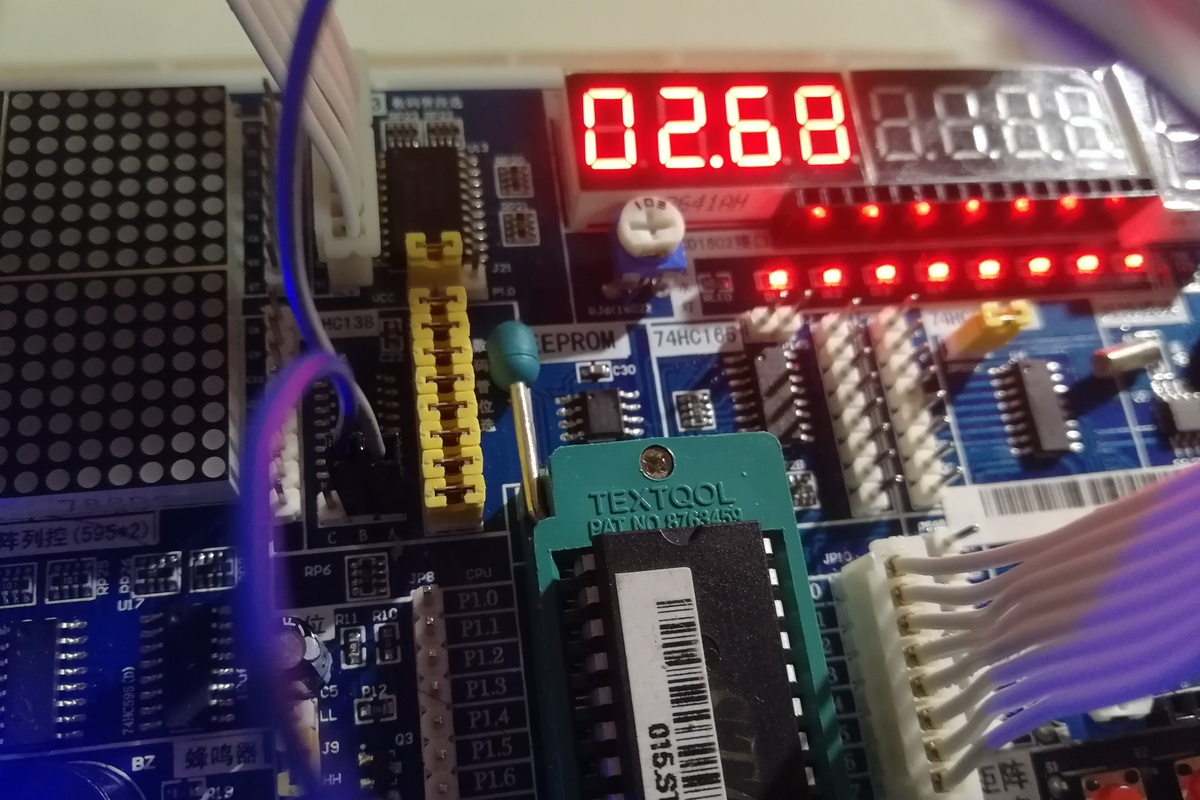• I/O 口控制实验
• 定时器实验
• 键盘接口实验
• 1-Wire 与 I2C 总线实验

## I/O 口控制实验

### 基础型

 `````` 1 2 3 4 5 6 7 8 9 10 11 12 13 14 15 16 17 18 19 20 21 22 23 24 25 `````` ``````#include #include #define uchar unsigned char #define uint unsigned int void delayms(uint k) { uint i; uchar j; for(i=k;i>0;i--) for(j=110;j>0;j--); } void main(){ char data s,i; while(1){ s=0xaa; P2=s; delayms(1000); for(i=0;i<7;i++){ s=_cror_(s,1); P2=s; delayms(1000); } } } ``````

 `````` 1 2 3 4 5 6 7 8 9 10 11 12 13 14 15 16 17 18 19 20 21 22 23 24 25 `````` ``````#include #include #define uchar unsigned char #define uint unsigned int void delayms(uint k) { uint i; uchar j; for(i=k;i>0;i--) for(j=110;j>0;j--); } void main(){ char data s,i; while(1){ s=0x80; P2=s; delayms(1000); for(i=0;i<7;i++){ s=_cror_(s,1); P2=s; delayms(1000); } } } ``````

### 设计型

 `````` 1 2 3 4 5 6 7 8 9 10 11 12 13 14 15 16 17 18 19 20 21 22 23 24 25 26 27 28 29 30 31 32 33 34 35 36 37 38 39 40 41 42 43 44 45 46 47 48 49 50 51 52 53 54 55 56 57 58 59 60 61 62 63 64 65 66 67 68 69 70 71 72 73 74 75 76 77 78 79 80 81 82 83 84 85 86 87 88 89 90 91 92 93 94 95 96 97 98 99 100 101 102 103 104 105 106 107 108 109 110 111 112 113 114 115 116 117 118 119 120 121 122 123 124 125 126 `````` ``````#include #include #define uchar unsigned char #define uint unsigned int void delay(uint k); void key1(); void key2(); void key3(); void key4(); sbit k1=P0^0; sbit k2=P0^1; sbit k3=P0^2; sbit k4=P0^3; void main() { while(1){ key1(); key2(); key3(); key4(); } } void delay(uint k) { uint i; uchar j; for(i=k;i>0;i--) for(j=55;j>0;j--){ if(k1==0){ P2=0x00; p=1; break; } else if(k2==0){ P2=0xff; p=1; break; } else if(k3==0){ key3(); } else if(k4==0){ key4(); } } } void key1() { if(k1==0){ P2=0x00; while(!k1); } } void key2() { if(k2==0){ P2=0xff; while(!k2); } } void key3() { if(k3==0){ char data s,i; int p=0,k=0; while(1){ s=0x80; P2=s; delay(1000); for(i=0;i<7;i++){ if(k1==0){ P2=0x00; p=1; break; } else if(k2==0){ P2=0xff; p=1; break; } s=_cror_(s,1); P2=s; delay(1000); } if(p==1) break; } while(!k3); } } void key4() { if(k4==0){ char data s,i; int p=0; while(1){ s=0xaa; P2=s; delay(1000); for(i=0;i<7;i++){ if(k1==0){ P2=0x00; p=1; break; } else if(k2==0){ P2=0xff; p=1; break; } s=_cror_(s,1); P2=s; delay(1000); } if(p==1) break; } while(!k4); } } ``````

### 探究型

 `````` 1 2 3 4 5 6 7 8 9 10 11 12 13 14 15 16 17 18 19 20 21 22 23 24 25 26 27 28 29 30 31 32 33 34 `````` ``````#include #include #define uchar unsigned char #define uint unsigned int void delay(uint k) { uint i; uchar j; for(i=k;i>0;i--) for(j=110;j>0;j--) ; } void main(){ P2=0xdb; delay(1000); P2=0xb7; delay(1000); P2=0x6f; delay(1000); while(1){ P2=0x7f; delay(500); P2=0xbf; delay(500); P2=0xdf; delay(500); P2=0xef; delay(500); P2=0xf7; delay(500); P2=0xfb; delay(500); } } ``````

 `````` 1 2 3 4 5 6 7 8 9 10 11 12 13 14 15 16 17 18 19 20 21 22 23 24 25 26 27 28 29 30 31 32 33 34 35 36 37 38 39 40 41 42 43 44 45 46 47 48 49 50 51 52 53 `````` ``````#include #include #define uchar unsigned char #define uint unsigned int sbit rt1=P0^0; //gre sbit rt2=P0^1; //red sbit up1=P2^0; //red sbit up2=P2^1; //gre sbit red1=P2^2; sbit yel1=P2^3; sbit gre1=P2^4; sbit red2=P2^5; sbit yel2=P2^6; sbit gre2=P2^7; //这里大部分定义并没有用到，只是为了标记每个颜色的灯对应的接口 void delay(uint k) { uint i; uchar j; for(i=k;i>0;i--) for(j=110;j>0;j--) ; } void main(){ int i; rt1=0; P2=0x7a; delay(2000); for(i=1;i<=3;i++){ gre2=1; delay(500); gre2=0; delay(500); } P2=0xba; delay(2000); P2=0xcd; rt1=1; rt2=0; delay(2000); for(i=1;i<=3;i++){ gre1=1; delay(500); gre1=0; delay(500); } P2=0xd5; delay(2000); rt2=1; up2=0; } ``````

## 定时器实验

### 基础型

 `````` 1 2 3 4 5 6 7 8 9 10 11 12 13 14 15 16 17 18 19 20 21 22 23 24 25 26 27 28 `````` ``````#include"reg52.h" #include"intrins.h" typedef unsigned char u8; typedef unsigned int u16; sbit led=P0^0; u16 i=0; void main() { TMOD=0x01; TH0=0XFC; TL0=0X18; ET0=1; EA=1; TR0=1; while(1); } void time0()interrupt 1 { TH0=0XFC; TL0=0X18; i++; if(i==1000) { i=0; led=~led; } } ``````

≈10软件编写
256定时器工作方式 2（8 位定时方式）
65536定时器工作方式 1（16 位定时方式）

### 设计型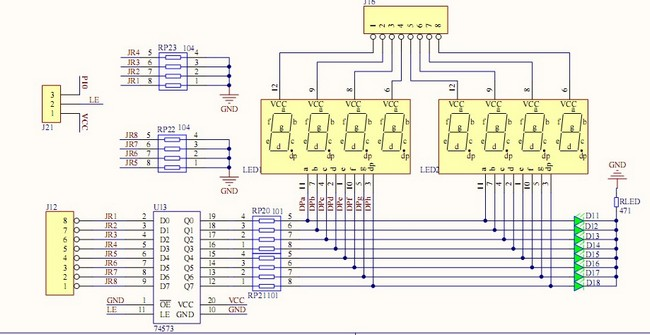◎ 动态数码管电路图

 `````` 1 2 3 4 5 6 7 8 9 10 11 12 13 14 15 16 17 18 19 20 21 22 23 24 25 26 27 28 29 30 31 32 33 34 35 36 37 38 39 40 41 42 43 44 45 46 47 48 49 50 51 52 53 54 55 56 57 58 59 60 61 62 63 64 65 66 67 68 69 70 71 72 73 74 75 76 77 78 79 80 81 82 83 84 85 `````` ``````#include sbit LS138A = P2^2; sbit LS138B = P2^3; sbit LS138C = P2^4; sbit k1=P2^0;//start sbit k2=P2^1;//return 60s unsigned int i; unsigned int a=6000; unsigned int LedNumVal=6000; unsigned char code Disp_Tab[] = {0x3f,0x06,0x5b,0x4f,0x66,0x6d,0x7d,0x07,0x7f,0x6f,0x40}; unsigned int LedOut; void main() { TMOD=0x01; TH0=0XFC; TL0=0X18; ET0=1; EA=0; TR0=1; while(1){ EA=1; LedOut=Disp_Tab[a%10000/1000]; LedOut=Disp_Tab[a%1000/100]|0x80; // 增加小数点，倒计时功能中包含小数 LedOut=Disp_Tab[a%100/10]; LedOut=Disp_Tab[a%10]; if(k1==0){ LedNumVal=6000; break; } } // 这里实现的是只有当按键按下才开始计数，而不是接通电源直接计数 while(1){ LedOut=Disp_Tab[LedNumVal%10000/1000]; LedOut=Disp_Tab[LedNumVal%1000/100]|0x80; LedOut=Disp_Tab[LedNumVal%100/10]; LedOut=Disp_Tab[LedNumVal%10]; if(k2==0){ EA=1; break; } } } void delay(unsigned int i) { char j; for(i; i>0; i--) for(j=200; j>0; j--); } void time0()interrupt 1 { TH0=0XFC; TL0=0X18; i++; if(i%4==0){ P0 = LedOut; LS138A=0; LS138B=0; LS138C=0; } else if(i%4==1){ P0 = LedOut; LS138A=1; LS138B=0; LS138C=0; } else if(i%4==2){ P0 = LedOut; LS138A=0; LS138B=1; LS138C=0; } else if(i%4==3){ P0 = LedOut; LS138A=1; LS138B=1; LS138C=0; } // 这里与其他同学设计的不同，其他人是直接连接单片机上数码管位码判定的 8 根管脚，需要用到 8 根线，我利用译码器，实现只用 3 根线控制 8 位显示。 if(i==10&&LedNumVal!=0){ i=0; --LedNumVal; } if(LedNumVal==0){ LedNumVal=0; } } ``````

### 探究型

 `````` 1 2 3 4 5 6 7 8 9 10 11 12 13 14 15 16 17 18 19 20 21 22 23 24 25 26 27 28 29 `````` ``````#include"reg52.h" #include"intrins.h" unsigned int i=0; unsigned int a=0; sbit led=P0^0; void main() { TMOD=0x01; TH0=0XFC; TL0=0X18; ET0=1; EA=1; TR0=1; while(1); } void time0()interrupt 1 { TH0=0XFC; TL0=0X18; i++; if(i>=2*(a%9)){ led=1; } if(i==20){ led=0; i=0; a++; } } ``````

ULN2003 模块电路图以及所用到的大功率 LED 如下图所示。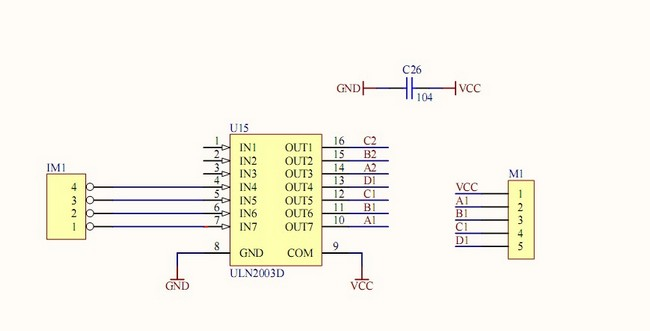◎ ULN2003 模块电路图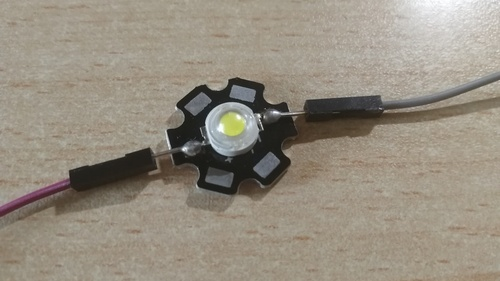◎ 大功率 LED

 `````` 1 2 3 4 5 6 7 8 9 10 11 12 13 14 15 16 17 18 19 20 21 22 23 24 25 26 27 28 29 30 31 32 33 34 35 36 37 38 39 40 41 42 43 44 45 46 47 48 49 50 51 52 53 54 55 56 57 58 59 60 61 62 63 64 65 66 67 68 69 70 71 72 73 74 75 76 77 `````` ``````#include"reg52.h" #include"intrins.h" unsigned int i=0; int a=0; unsigned int d; unsigned int p; unsigned int LedNumVal; unsigned char code Disp_Tab[] = {0x3f,0x06,0x5b,0x4f,0x66,0x6d,0x7d,0x07,0x7f,0x6f,0x40}; unsigned int LedOut; sbit led=P0^0; sbit k1=P1^0; // + sbit k2=P1^1; // - void key1(); void key2(); void delay(unsigned int k) { for(d=k;d>0;d--) for(p=110;p>0;p--) ; } void main() { TMOD=0x01; TH0=0XFC; TL0=0X18; ET0=1; EA=1; TR0=1; while(1){ LedNumVal=a; LedOut=Disp_Tab[LedNumVal]; P2=LedOut; } } void time0()interrupt 1 { TH0=0XFC; TL0=0X18; i++; if(i>=2*a){ led=1; } if(i==20){ led=0; i=0; } key1(); key2(); } void key1() { if(k1==0){ delay(50); if(k1==0){ led=1; a--; if(a<0){ a=9; } while(!k1); } } } void key2() { if(k2==0){ delay(50); if(k2==0){ led=1; a++; if(a>9){ a=0; } while(!k2); } } } ``````

## 键盘接口实验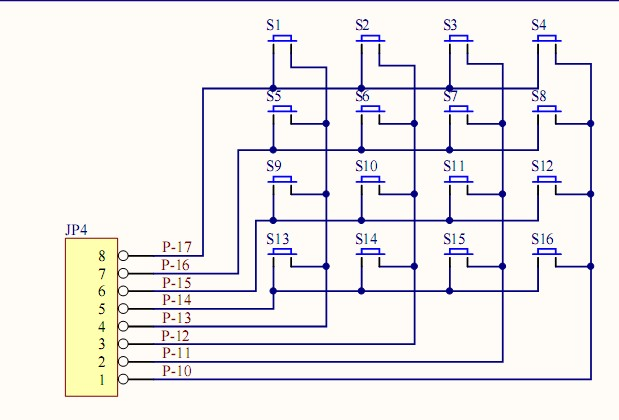◎ 矩阵键盘电路图

### 基础型

 `````` 1 2 3 4 5 6 7 8 9 10 11 12 13 14 15 16 17 18 19 20 21 22 23 24 25 26 27 28 29 30 31 32 `````` ``````#include"reg52.h" #include"intrins.h" unsigned int i=0; unsigned int a=0; unsigned char code Disp_Tab[] = {0x88,0x83,0xC6,0xA1,0x86,0x8e}; // 对应字母a、b、c、d、e、f void main() { TMOD=0x01; TH0=0XFC; TL0=0X18; ET0=1; EA=1; TR0=1; while(1); } void time0()interrupt 1 { TH0=0XFC; TL0=0X18; i++; if(i<100) { P0=Disp_Tab[a]; } if(i==1000){ a++; i=0; } if(a==6) a=0; } ``````

 `````` 1 2 3 4 5 6 7 8 9 10 11 12 13 14 15 16 17 18 19 20 21 22 23 24 25 26 27 28 29 30 31 32 33 34 35 36 37 38 39 40 41 42 43 44 45 46 47 48 49 50 51 52 53 54 55 56 57 58 59 60 61 62 63 64 65 66 67 68 69 70 71 72 73 74 `````` ``````#include #define uchar unsigned char #define uint unsigned int uchar dis_buf; uchar temp; uchar key; void delay(uint k); uchar code LED7Code[] = {~0x3F,~0x06,~0x5B,~0x4F,~0x66,~0x6D,~0x7D,~0x07,~0x7F,~0x6F,~0x77,~0x7C,~0x39,~0x5E,~0x79,~0x71}; void delay(uint k) { uint i; uchar j; for(i=k;i>0;i--) for(j=110;j>0;j--); } void keyscan(void) { temp = 0; P1=0xF0; delay(50); temp=P1; temp=temp&0xF0; temp=~((temp>>4)|0xF0); if(temp==1) key=0; else if(temp==2) key=1; else if(temp==4) key=2; else if(temp==8) key=3; else key = 16; P1=0x0F; delay(50); temp=P1; temp=temp&0x0F; temp=~(temp|0xF0); if(temp==1) key=key+0; else if(temp==2) key=key+4; else if(temp==4) key=key+8; else if(temp==8) key=key+12; else key = 16; dis_buf = key; dis_buf = dis_buf & 0x0f; } void keydown(void) { P1=0xF0; if(P1!=0xF0) { keyscan(); } } main() { P0=0xFF; P1=0xFF; delay(50); while(1){ keydown(); P0 = LED7Code[dis_buf%16]; } } ``````

### 设计型

 `````` 1 2 3 4 5 6 7 8 9 10 11 12 13 14 15 16 17 18 19 20 21 22 23 24 25 26 27 28 29 30 31 32 33 34 35 36 37 38 39 40 41 42 43 44 45 46 47 48 49 50 51 52 53 54 55 56 57 58 59 60 61 62 63 64 65 66 67 68 69 70 71 72 73 74 75 76 77 78 79 `````` ``````#include sbit LS138A = P2^2; sbit LS138B = P2^3; sbit LS138C = P2^4; unsigned int i; unsigned int a=0; unsigned char code Disp_Tab[] = {0x3f,0x06,0x5b,0x4f,0x66,0x6d,0x7d,0x07,0x7f,0x00}; // 0 1 2 3 4 5 6 7 8 9 unsigned int num={9,9,9,3,1,7,0,0,0,0,0,0,0,9,9,9,9,3,1,7,0}; // 在这个数组中写入自己的学号或任何想要显示的数字，格式是在循环到末尾的 10 位数后，重新回到第一位开启新一轮的循环。9 表示什么都不显示。 unsigned int LedOut; void main() { TMOD=0x01; TH0=0XFC; TL0=0X18; ET0=1; EA=1; TR0=1; while(1){ LedOut=Disp_Tab[num[a]]; LedOut=Disp_Tab[num[a+1]]; LedOut=Disp_Tab[num[a+2]]; LedOut=Disp_Tab[num[a+3]]; LedOut=Disp_Tab[num[a+4]]; LedOut=Disp_Tab[num[a+5]]; LedOut=Disp_Tab[num[a+6]]; LedOut=Disp_Tab[num[a+7]]; } } void time0()interrupt 1 { TH0=0XFC; TL0=0X18; i++; if(i%8==0){ P3=LedOut; LS138A=0; LS138B=0; LS138C=0; } else if(i%8==1){ P3=LedOut; LS138A=1; LS138B=0; LS138C=0; } else if(i%8==2){ P3=LedOut; LS138A=0; LS138B=1; LS138C=0; } else if(i%8==3){ P3=LedOut; LS138A=1; LS138B=1; LS138C=0; } else if(i%8==4){ P3=LedOut; LS138A=0; LS138B=0; LS138C=1; } else if(i%8==5){ P3=LedOut; LS138A=1; LS138B=0; LS138C=1; } else if(i%8==6){ P3=LedOut; LS138A=0; LS138B=1; LS138C=1; } else if(i%8==7){ P3=LedOut; LS138A=1; LS138B=1; LS138C=1; } if(i==500){ a++; i=0; if(a==14) a=0; } } ``````

### 探究型

 `````` 1 2 3 4 5 6 7 8 9 10 11 12 13 14 15 16 17 18 19 20 21 22 23 24 25 26 27 28 29 30 31 32 33 34 35 36 37 38 39 40 41 42 43 44 45 46 47 48 49 50 51 52 53 54 55 56 57 58 59 60 61 62 63 64 65 66 67 68 69 70 71 72 73 74 75 76 77 78 79 80 81 82 83 84 85 86 87 88 89 90 91 92 93 94 95 96 97 98 99 100 101 102 103 104 105 106 107 108 109 110 111 112 113 114 115 116 117 118 119 120 121 122 123 124 125 126 127 128 129 130 131 132 133 134 135 136 137 138 139 140 141 142 143 144 145 146 147 148 149 150 151 152 153 154 155 156 157 158 159 `````` ``````#include sbit LS138A = P2^2; sbit LS138B = P2^3; sbit LS138C = P2^4; unsigned int i; unsigned int sec=00; unsigned int min=00; unsigned int hou=00; unsigned char code Disp_Tab[] = {0x3f,0x06,0x5b,0x4f,0x66,0x6d,0x7d,0x07,0x7f,0x6f,0x40}; unsigned int LedOut; unsigned char temp; unsigned char key=0; void delay(unsigned int k); void keyscan(void); void keydown(void); void main() { TMOD=0x01; TH0=0XFC; TL0=0X18; ET0=1; EA=1; TR0=1; while(1){ keydown(); LedOut=Disp_Tab[hou%100/10]; LedOut=Disp_Tab[hou%10]; LedOut=Disp_Tab[min%100/10]; LedOut=Disp_Tab[min%10]; //LedOut=Disp_Tab[sec%10000/1000]; //LedOut=Disp_Tab[sec%1000/100]|0x80; LedOut=Disp_Tab[sec%100/10]; LedOut=Disp_Tab[sec%10]; } } void time0()interrupt 1 { TH0=0XFC; TL0=0X18; i++; if(i%8==0){ P3=LedOut; LS138A=0; LS138B=0; LS138C=0; } else if(i%8==1){ P3=LedOut; LS138A=1; LS138B=0; LS138C=0; } else if(i%8==2){ P3=LedOut; LS138A=0; LS138B=1; LS138C=0; } else if(i%8==3){ P3 = LedOut; LS138A=1; LS138B=1; LS138C=0; } else if(i%8==4){ P3=LedOut; LS138A=0; LS138B=0; LS138C=1; } else if(i%8==5){ P3=LedOut; LS138A=1; LS138B=0; LS138C=1; } else if(i%8==6){ P3=LedOut; LS138A=0; LS138B=1; LS138C=1; } else if(i%8==7){ P3=LedOut; LS138A=1; LS138B=1; LS138C=1; } if(i==1000){ i=0; sec++; if(sec==60){ sec=0; min++; } if(min==60){ min=0; hou++; } if(hou==24){ hou=0; } } } void delay(unsigned int k) { unsigned int m; unsigned char n; for(m=k;m>0;m--) for(n=110;n>0;n--); } void keyscan(void) { temp=0; P1=0xF0; delay(50); temp=P1; temp=temp&0xF0; temp=~((temp>>4)|0xF0); if(temp==1) key=0; else if(temp==2) key=1; else if(temp==4) key=2; else if(temp==8) key=3; else key = 16; P1=0x0F; delay(50); temp=P1; temp=temp&0x0F; temp=~(temp|0xF0); if(temp==1) key=key+0; else if(temp==2) key=key+4; else if(temp==4) key=key+8; else if(temp==8) key=key+12; else key = 16; if(key==0){ sec++; if(sec==60) sec=0; } else if(key==1){ min++; if(min==60) min=0; } else if(key==2){ hou++; if(hou==24) hou=0; } } void keydown(void) { P1=0xF0; if(P1!=0xF0) { keyscan(); } } ``````

 `````` 1 2 3 4 5 6 7 8 9 10 11 12 13 14 15 16 17 18 19 20 21 22 23 24 25 26 27 28 29 30 31 32 33 34 35 36 37 38 39 40 41 42 43 44 45 46 47 48 49 50 51 52 53 54 55 56 57 58 59 60 61 62 63 64 65 66 67 68 69 70 71 72 73 74 75 76 77 78 79 80 81 82 83 84 85 86 87 88 89 90 91 92 93 94 95 96 97 98 99 100 `````` ``````#include sbit LS138A = P2^2; sbit LS138B = P2^3; sbit LS138C = P2^4; unsigned int i; unsigned int a=0; unsigned char code Disp_Tab[] = {0x39, 0x09, 0x0f, 0x01, 0x08, 0x38, 0x19, 0x29, 0x31, 0x0e, 0x0d, 0x0b, 0x07}; unsigned int code num={5,1,1,1,1,1,1,2, 6,1,1,1,1,1,1,2, 7,1,1,1,1,1,1,2, 8,1,1,1,1,1,1,2, 0,3,1,1,1,1,1,2, 0,1,3,1,1,1,1,2, 0,1,1,3,1,1,1,2, 0,1,1,1,3,1,1,2, 0,1,1,1,1,3,1,2, 0,1,1,1,1,1,3,2, 0,1,1,1,1,1,1,12, 0,1,1,1,1,1,1,11, 0,1,1,1,1,1,1,10, 0,1,1,1,1,1,1,9, 0,1,1,1,1,1,4,2, 0,1,1,1,1,4,1,2, 0,1,1,1,4,1,1,2, 0,1,1,4,1,1,1,2, 0,1,4,1,1,1,1,2, 0,4,1,1,1,1,1,2}; unsigned int LedOut; void main() { TMOD=0x01; TH0=0XFC; TL0=0X18; ET0=1; EA=1; TR0=1; while(1){ LedOut=Disp_Tab[num[a]]; LedOut=Disp_Tab[num[a+1]]; LedOut=Disp_Tab[num[a+2]]; LedOut=Disp_Tab[num[a+3]]; LedOut=Disp_Tab[num[a+4]]; LedOut=Disp_Tab[num[a+5]]; LedOut=Disp_Tab[num[a+6]]; LedOut=Disp_Tab[num[a+7]]; } } void time0()interrupt 1 { TH0=0XFC; TL0=0X18; i++; if(i%8==0){ P3=LedOut; LS138A=0; LS138B=0; LS138C=0; } else if(i%8==1){ P3=LedOut; LS138A=1; LS138B=0; LS138C=0; } else if(i%8==2){ P3=LedOut; LS138A=0; LS138B=1; LS138C=0; } else if(i%8==3){ P3 = LedOut; LS138A=1; LS138B=1; LS138C=0; } else if(i%8==4){ P3=LedOut; LS138A=0; LS138B=0; LS138C=1; } else if(i%8==5){ P3=LedOut; LS138A=1; LS138B=0; LS138C=1; } else if(i%8==6){ P3=LedOut; LS138A=0; LS138B=1; LS138C=1; } else if(i%8==7){ P3=LedOut; LS138A=1; LS138B=1; LS138C=1; } if(i==500){ a=a+8; i=0; if(a==160) a=0; } } ``````

## 1-Wire 与 I2C 总线实验

### 基础型

DS18B20 原理图：◎ DS18B20

 `````` 1 2 3 4 5 6 7 8 9 10 11 12 13 14 15 16 17 18 19 20 21 22 23 24 25 26 27 28 29 30 31 32 33 34 35 36 37 38 39 40 41 42 43 44 45 46 47 48 49 50 51 52 53 54 55 56 57 58 59 60 61 62 63 64 65 66 67 68 69 70 71 72 73 74 75 76 77 78 79 80 81 82 83 84 85 86 87 88 89 90 91 92 93 94 95 96 97 98 99 100 101 102 103 104 105 106 107 108 109 110 111 112 113 `````` ``````#include #define uchar unsigned char #define uint unsigned int sbit dq=P3^7; uint PuZh; uchar sb={'0','1','2','3','4','5','6','7','8','9'}; // 至于为什么把这个数组名字定义为 sb，还有下面的 ssb，可能是当时的心情有些烦躁吧 void UsartConfiguration(); void Delay10ms(uint c); void sdelay(uint i) { while(i--); } void DS18B20_Reset() { uchar x=0; dq=1; sdelay(8); dq=0; sdelay(80); dq=1; sdelay(14); sdelay(20); } void DS18B20_Write_Byte(uchar dat) { uchar i=0; for(i=8;i>0;i--){ dq=0; dq=dat&0x01; sdelay(5); dq=1; dat>>=1; } } uchar DS18B20_Read_Byte() { uchar i=0,dat=0; for(i=8;i>0;i--){ dq=0; dat>>=1; dq=1; if(dq) dat|=0x80; sdelay(4); } return(dat); } uint GET_Temperature() { uchar a=0,b=0; uint t=0; float tt=0; DS18B20_Reset(); DS18B20_Write_Byte(0xcc); DS18B20_Write_Byte(0x44); DS18B20_Reset(); DS18B20_Write_Byte(0xcc); DS18B20_Write_Byte(0xbe); a=DS18B20_Read_Byte(); b=DS18B20_Read_Byte(); t=b; t<<=8; t=t|a; tt=t*0.0625; t=tt*10+0.5; return(t); } void main() { uchar i; uint x; uint y,z; uchar ssb; UsartConfiguration(); while(1){ PuZh=GET_Temperature(); x=PuZh/100; y=PuZh%100%10; z=PuZh%100/10; ssb=sb[x]; ssb=sb[y]; ssb='.'; ssb=sb[z]; ssb=' '; for(i=0;i<5;i++){ SBUF=ssb[i]; while(!TI); TI=0; } Delay10ms(50); } } void UsartConfiguration() { SCON=0X50; TMOD=0X20; PCON=0X80; TH1=0XF3; TL1=0XF3; TR1=1; } void Delay10ms(unsigned int c) { unsigned char a,b; for(;c>0;c--){ for(b=38;b>0;b--){ for(a=130;a>0;a--); } } } ``````

### 设计型

PCF8591 原理图：◎ PCF8591

`i2c.h`

 `````` 1 2 3 4 5 6 7 8 9 10 11 12 13 14 15 `````` ``````// i2c.h #ifndef __I2C_H_ #define __I2C_H_ #include sbit SCL=P2^1; sbit SDA=P2^0; unsigned char I2C_ReadByte(); void I2C_SendByte( unsigned char dat); void I2C_Stop(); void I2C_Start(); #endif ``````

`i2c.c`

 `````` 1 2 3 4 5 6 7 8 9 10 11 12 13 14 15 16 17 18 19 20 21 22 23 24 25 26 27 28 29 30 31 32 33 34 35 36 37 38 39 40 41 42 43 44 45 46 47 48 49 50 51 52 53 54 55 56 57 58 59 60 61 62 63 64 65 66 67 68 69 70 71 72 `````` ``````// i2c.c #include "i2c.h" #include #define uchar unsigned char #define uint unsigned int #define somenop() _nop_(),_nop_(),_nop_(),_nop_(),_nop_(),_nop_() void I2C_Start() { SCL=1; _nop_();_nop_();_nop_();_nop_();_nop_(); SDA=1; _nop_();_nop_();_nop_();_nop_();_nop_(); SDA=0; _nop_();_nop_();_nop_();_nop_();_nop_(); SCL=0; _nop_();_nop_();_nop_();_nop_();_nop_(); } void I2C_Stop() { SDA=0; _nop_();_nop_();_nop_();_nop_();_nop_(); SCL=1; _nop_();_nop_();_nop_();_nop_();_nop_(); SDA=1; _nop_();_nop_();_nop_();_nop_();_nop_(); } void I2C_SendByte(unsigned char dat) { uchar i,j,b=0; for(i=0;i<8;i++){ SCL=0; _nop_();_nop_();_nop_();_nop_();_nop_(); SDA=(bit)(dat&0x80); dat<<=1; SCL=1; _nop_();_nop_();_nop_();_nop_();_nop_(); } SCL=0; _nop_();_nop_();_nop_();_nop_();_nop_(); SDA=1; _nop_();_nop_();_nop_();_nop_();_nop_(); SCL=1; _nop_(); while((SDA==1)&&(j<250)) j++; SCL=0; _nop_(); } unsigned char I2C_ReadByte() { uchar i,dat=0; SCL=0; _nop_();_nop_();_nop_();_nop_();_nop_(); SDA=1; _nop_(); for(i=0;i<8;i++){ SCL=1; _nop_();_nop_();_nop_();_nop_();_nop_(); dat<<=1; if(SDA==1) dat=dat|0x01; _nop_();_nop_();_nop_();_nop_();_nop_(); SCL=0; // 下降沿时读取数据 _nop_();_nop_();_nop_();_nop_();_nop_(); } return dat; } ``````

`main.c`

 `````` 1 2 3 4 5 6 7 8 9 10 11 12 13 14 15 16 17 18 19 20 21 22 23 24 25 26 27 28 29 30 31 32 33 34 35 36 37 38 39 40 41 42 43 44 45 46 47 48 49 50 51 52 53 54 55 56 57 58 59 60 61 62 63 64 65 66 67 68 69 70 `````` ``````// main.c #include #include #include #define uint unsigned int #define uchar unsigned char #define WRITEADDR 0x90 #define READADDR 0x91 void Pcf8591SendByte(uchar channel) { I2C_Start(); I2C_SendByte(WRITEADDR); I2C_SendByte(0x40|channel); I2C_Stop(); } uchar Pcf8591ReadByte() { uchar num; I2C_Start(); I2C_SendByte(READADDR); num=I2C_ReadByte(); I2C_Stop(); return num; } void UsartInit() { SCON=0X50; TMOD=0X20; PCON=0X80; TH1=0XF3; TL1=0XF3; TR1=1; } void delay1s(void) { unsigned char a,b,c; for(c=167;c>0;c--) for(b=171;b>0;b--) for(a=16;a>0;a--); _nop_(); } void main() { uint adNum,i,value; uchar PuZh; UsartInit(); while(1){ Pcf8591SendByte(0); adNum=Pcf8591ReadByte(); value=adNum; value=100-value*100/255; PuZh=32; PuZh=value/10+48; PuZh=value%10+48; PuZh=32; for(i=0;i<4;i++){ SBUF=PuZh[i]; while(!TI); TI=0; } delay1s(); } } ``````

### 探究型

 `````` 1 2 3 4 5 6 7 8 9 10 11 12 13 14 15 16 17 18 19 20 21 22 23 24 25 26 27 28 29 30 31 32 33 34 35 36 37 38 39 40 41 42 43 44 45 46 47 48 49 50 51 52 53 54 55 56 57 58 59 60 61 62 63 64 65 66 67 68 69 70 71 72 73 74 75 76 77 78 79 80 81 82 83 84 85 86 87 88 89 90 91 92 93 94 95 96 97 98 99 100 101 102 103 104 105 106 107 108 109 110 111 112 113 114 115 116 117 118 119 120 121 122 123 124 125 126 127 128 129 130 131 132 133 134 135 136 137 138 139 140 141 142 143 144 145 146 147 148 149 150 151 152 153 154 155 156 157 158 159 160 161 162 163 164 165 166 167 168 169 170 `````` ``````#include #include #define uchar unsigned char #define uint unsigned int sbit sda=P2^0; sbit scl=P2^1; uchar receiveData; void UsartConfiguration(); void delay() //5us {;;} void delay1(uint z)//1ms { uint x,y; for(x=z;x>0;x--) for(y=120;y>0;y--); } void start() { scl=1; _nop_(); sda=1; delay(); sda=0; delay(); scl=0; _nop_(); } void stop() { scl=1; _nop_(); sda=0; delay(); sda=1; delay(); } void respons() { scl=1; _nop_(); sda=0; delay(); scl=0; _nop_(); } void norespons() { scl=1; _nop_(); sda=1; delay(); } void init() { scl=1; _nop_(); sda=1; _nop_(); } void write_byte(uchar dat) { uchar i,temp; temp=dat; for(i=0;i<8;i++){ scl=0; _nop_(); if((temp&0x80)==0x80) sda=1; else sda=0; scl=1; _nop_(); temp<<=1; } scl=0; _nop_(); } uchar read_byte() { uchar dat,i; sda=1; _nop_(); scl=0; _nop_(); for(i=0;i<8;i++){ scl=1; _nop_(); if(sda) dat|=0x01; if(i<7) dat<<=1; scl=0; _nop_(); } return dat; } void DAC_write(uchar dat) { start(); write_byte(0x90); respons(); write_byte(0x40); respons(); write_byte(dat); respons(); stop(); } uchar ADC_read(uchar com) { uchar dat; dat=com; start(); write_byte(0x90); respons(); write_byte(com); respons(); start(); write_byte(0x91); respons(); dat=read_byte(); norespons(); stop(); return dat; } void main() { uchar ss=0,ad_value; uint aa; init(); UsartConfiguration(); while(1){ if(receiveData=='0') aa=0; else if(receiveData=='1') aa=100; else if(receiveData=='2') aa=110; else if(receiveData=='3') aa=120; else if(receiveData=='4') aa=130; else if(receiveData=='5') aa=140; else if(receiveData=='6') aa=150; else if(receiveData=='7') aa=160; else if(receiveData=='8') aa=170; else if(receiveData=='9') aa=180; ad_value=ADC_read(0x42); DAC_write(aa); delay1(20); } } void UsartConfiguration() { SCON=0X50; TMOD=0X20; PCON=0X80; TH1=0XF3; TL1=0XF3; ES=1; EA=1; TR1=1; } void Usart() interrupt 4 { receiveData=SBUF; RI=0; SBUF=receiveData; while(!TI); TI=0; } ``````